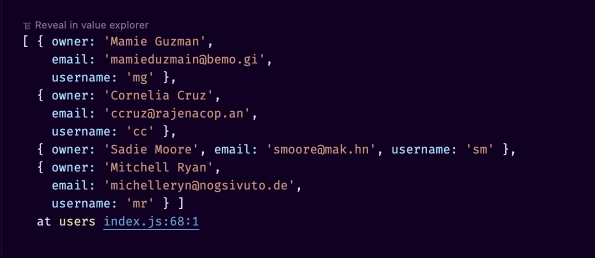# const - JS | lectureWorking With Arrays

The map Method in Practice

In this lecture, we will practice a little bit more how to use the map and the `forEach` methods. So let's consider the below four user accounts.

script.js
``````const users = [
{
owner: 'Mamie Guzman',
email: 'mamieduzmain@bemo.gi',
},
{
owner: 'Cornelia Cruz',
email: 'ccruz@rajenacop.an',
},
{
email: 'smoore@mak.hn',
},
{
owner: 'Mitchell Ryan',
email: 'michelleryn@nogsivuto.de',
},
];``````

Now let's say we want to compute usernames for each of the account owners. And the username is simply the initials of each user. Let's just start with a single string to make it easier to understand. Then we will generalize it for the entire array.

Let's consider the below user:

script.js
``const user = 'Virginia Chambers';``

The username of the above user should be `vc`. That is, just the initials of the user all in lowercase. Hence, let's do that.

The first thing we will do is to convert the user to lowercase.

script.js
``const username = user.toLowerCase(); // 'virginia chambers'``

Now, what we need to do is to take the first letter of each word in the username. To do that, we first need to split up the string into multiple words or names.

script.js
``const username = user.toLowerCase().split(' '); // ['virginia', 'chambers']``

Next, to take the first letter of each name or word in the array, we need to loop over it and then take the first letter in each iteration and add them to a new array. Then at the end, we join that array and end up with the username `vc`.

Let's do that!

So the first step is, looping over the array, then taking the first letter of each word and then putting it into a new array. If you remember, that's exactly what the map method does.

script.js
``````const username = user
.toLowerCase()
.split(' ')
.map((word) => word); // ['v', 'c']``````

Since the result of the above operation is an array, let's simply call the join method on it to get the final result.

script.js
``````const username = user
.toLowerCase()
.split(' ')
.map((word) => word)
.join(''); // 'vc'``````

Great! Now that we have the desired result, let's create a function that will do the above operation.

script.js
``````const createUsernames = (user) => {
.toLowerCase()
.split(' ')
.map((word) => word)
.join('');
};

Everything works as expected! But what we actually want is to compute one username for each user in our `users` array. So to do that, should we use the map or the forEach method? Well, we do not need to create a new array in this situation. All we want to do is to modify the object. i.e., the elements that already exist in the `users` array.

So, since all we need to do is to simply loop over the `users` array and then do something with each element, we need to go with the `forEach` method.

Let's start by updating our function. Because right now, we are only receiving one user instead of an array of users, we need to change that.

script.js
``````const createUsernames = (users) => {
users.forEach((user) => {
.toLowerCase()
.split(' ')
.map((word) => word)
.join('');
});
};``````

Note that we are not returning anything because again, what we're doing here is to produce a side effect. That is, we are modifying the original array. Hence, there is no need to return anything.

Now, let's call the function and see what happens.

script.js
``````createUsernames(users);
console.log(users);``````We indeed get the desired result!

In this lecture, it was very important to understand the use case for the map method here, which was perfect because it allowed us to create a new simple array that only contains the initials of whatever name it is used on. And then, on the other hand, the `forEach` method. It was a great use case to produce some so-called side effects. In other words, to simply do some work without returning anything.

Let's now move on to our next transforming method which is the `filter` method.